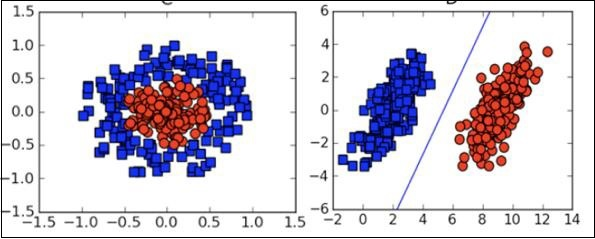# Machine Learning - Skills

Machine Learning has a very large width and requires skills across several domains. The skills that you need to acquire for becoming an expert in Machine Learning are listed below −

• Statistics
• Probability Theories
• Calculus
• Optimization techniques
• Visualization

## Necessity of Various Skills of Machine Learning

To give you a brief idea of what skills you need to acquire, let us discuss some examples −

### Mathematical Notation

Most of the machine learning algorithms are heavily based on mathematics. The level of mathematics that you need to know is probably just a beginner level. What is important is that you should be able to read the notation that mathematicians use in their equations. For example - if you are able to read the notation and comprehend what it means, you are ready for learning machine learning. If not, you may need to brush up your mathematics knowledge.

$$f_{AN}(net-\theta)=\begin{cases}\gamma & if\:net-\theta \geq \epsilon\\net-\theta & if - \epsilon< net-\theta <\epsilon\\ -\gamma & if\:net-\theta\leq- \epsilon\end{cases}$$

$$\displaystyle\\\max\limits_{\alpha}\begin{bmatrix}\displaystyle\sum\limits_{i=1}^m \alpha-\frac{1}{2}\displaystyle\sum\limits_{i,j=1}^m label^\left(\begin{array}{c}i\\ \end{array}\right)\cdot\:label^\left(\begin{array}{c}j\\ \end{array}\right)\cdot\:a_{i}\cdot\:a_{j}\langle x^\left(\begin{array}{c}i\\ \end{array}\right),x^\left(\begin{array}{c}j\\ \end{array}\right)\rangle \end{bmatrix}$$

$$f_{AN}(net-\theta)=\left(\frac{e^{\lambda(net-\theta)}-e^{-\lambda(net-\theta)}}{e^{\lambda(net-\theta)}+e^{-\lambda(net-\theta)}}\right)\;$$

### Probability Theory

Here is an example to test your current knowledge of probability theory: Classifying with conditional probabilities.

$$p(c_{i}|x,y)\;=\frac{p(x,y|c_{i})\;p(c_{i})\;}{p(x,y)\;}$$

With these definitions, we can define the Bayesian classification rule −

• If P(c1|x, y) > P(c2|x, y) , the class is c1 .
• If P(c1|x, y) < P(c2|x, y) , the class is c2 .

### Optimization Problem

Here is an optimization function

$$\displaystyle\\\max\limits_{\alpha}\begin{bmatrix}\displaystyle\sum\limits_{i=1}^m \alpha-\frac{1}{2}\displaystyle\sum\limits_{i,j=1}^m label^\left(\begin{array}{c}i\\ \end{array}\right)\cdot\:label^\left(\begin{array}{c}j\\ \end{array}\right)\cdot\:a_{i}\cdot\:a_{j}\langle x^\left(\begin{array}{c}i\\ \end{array}\right),x^\left(\begin{array}{c}j\\ \end{array}\right)\rangle \end{bmatrix}$$

Subject to the following constraints −

$$\alpha\geq0,and\:\displaystyle\sum\limits_{i-1}^m \alpha_{i}\cdot\:label^\left(\begin{array}{c}i\\ \end{array}\right)=0$$

If you can read and understand the above, you are all set.

### Visualization

In many cases, you will need to understand the various types of visualization plots to understand your data distribution and interpret the results of the algorithm’s output.Besides the above theoretical aspects of machine learning, you need good programming skills to code those algorithms.

So what does it take to implement ML? Let us look into this in the next chapter.

## Useful Video Courses

Video

#### Theory of Machines

168 Lectures 13.5 hours

Video

#### Mathematics for Data Science and Machine Learning using R

64 Lectures 10.5 hours

Video

#### Python Machine Learning & Data Science for Dummies

91 Lectures 10 hours

Video

#### Linear Regression Analysis in R - Machine Learning Basics

54 Lectures 6 hours

Video

#### Logistic Regression, LDA & KNN in R: Machine Learning models

49 Lectures 5 hours

Video

#### Support Vector Machines (SVM) in Python

35 Lectures 4 hours